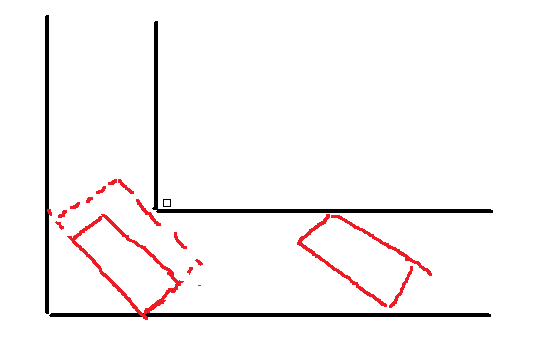# 题意与分析# 代码

/*
* ACM Code => cf99e.java
* Written by Sam X
* Date: 三月, 20, 2019
* Time: 14:25
*/
import java.util.*;
import java.math.*;

public class cf99e
{
static double a,b,l;

static final double eps=1e-9;
static final int sgn(double x)
{
if(Math.abs(x)<eps) return 0;
else if(x>eps) return 1;
else return -1;
}
static final double calc(double x)
{
return (Math.sqrt(l*l-x*x)*(b-x)+x*a)/l;
}

static void ternarySearch(double l, double r)
{
double midl, midr;
while(l+eps<r)
{
midl=l+(r-l)/3;
midr=r-(r-l)/3;
//System.out.println(mid+" "+midmid);
//System.out.println(calc(mid)+" "+calc(midmid));
{
r=midr;
}
else l=midl;
}
if(sgn(calc(l))<1)
else System.out.println(calc(l));
}
public static void main(String args[])
{
Scanner cin = new Scanner(System.in);
a=cin.nextDouble();
b=cin.nextDouble();
l=cin.nextDouble();
if(a-b>eps)
{
double t=a;
a=b;
b=t;
}
double minab = Math.min(a,b),
maxab = Math.max(a,b);
if(sgn(l-minab)<1) System.out.printf("%.7f\n", l);
else if(sgn(minab-l)<1 && sgn(l-maxab)<1) System.out.printf("%.7f\n", a);
else
{
ternarySearch(0, l);
}
cin.close();
}
}

posted @ 2019-03-20 16:14  SamHX  阅读(108)  评论(0编辑  收藏